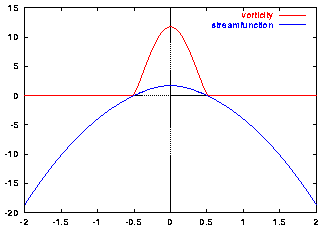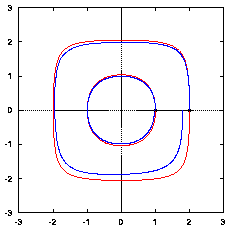## The Bessel monopole

The so-called Bessel monopole is a monopolar vortex structure with a continuous vorticity distribution within a circular region. This monopole has no net linear momentum, so it does not move. It has, however, a net angular momentum: it rotates about its centre, anti-clockwise (clockwise) for a positive (negative) vortex. It does this with constant velocity and without change of form. It is a stationary solution of the two-dimensional vorticity equation (Navier-Stokes equation) when there are no viscous effects and the domain is infinite.
When viscous effects play a role, the vorticity is spread over a larger area and the monopole decreases in strength, so that the rotation velocity also decreases in the course of time. In case the domain is finite, the velocity of the dipole is less than in an infinite domain.

### A somewhat more precise description

The Bessel monopole has a continuous vorticity distribution within a circle of radius a. Inside this circle there is a linear relationship between the vorticity omega and the streamfunction psi, namely: omega=k2psi, where k is a constant given by ak=2.40. The vorticity distribution has the form of a first order Bessel function (J0), hence its name
The flow outside the monopole is circular with an angular velocity of Gamma/2*pi*r, with r the radial distance to the centre of the vortex and Gamma the strength (circulation) of the monopole. This flow is irrotational: outside there is no vorticity: omega=0.

The vorticity distribution of this monopolar vortex can be seen in the following two pictures.A plot of contours of vorticity of a Bessel monopole with radius a=0.5 and strength Gamma=4 (the max. of vorticity is 11.8). Streamlines of this monopole coincide with contours of vorticity: the fluid rotates about the centre of the monopole, anti-clockwise in this positive monopole. Outside the monopole, where the vorticity equals zero, the fluid also rotates about the centre. Profile of vorticity (red) and streamfunction (blue) along a line through the centre of the monopole. The streamfunction is shown in a frame co-rotating with the monopole (rotation rate: -9.5).

### Finite domain

A monopolar vortex only rotates about its centre, without moving, in an infinite domain. If placed in a finite domain, however, the monopole feels its mirror images in the boundaries. When the monopole is not in the centre of the domain, it will start moving due to the "interaction" with the mirror images.
• For stress-free boundaries, this motion is around the centre. And if the fluid is inviscid, then the monopole will return to the point it started from at some moment in time. If the fluid is viscous, the monopole becomes weaker and will gradually decay and move closer to the centre of the domain. See the figure on the left below.

• For no-slip boundaries, where the velocity at the boundary equals zero, the monopole's motion is a spiral towards the centre of the domain. See the figure on the right below.Trajectories of a monopole starting from (1,0) or (2,0) in the shown domain with stress-free boundaries without viscosity (red) and with viscosity (blue; Re=1000) -- the monopole circles around the centre of the domain; only one revolution is shown. Trajectories in the same domain, but with no-slip boundaries in red (blue as in the left graph, for comparison) -- the monopole spirals to the origin.

For details on this, see:
Van Geffen, J.H.G.M., Meleshko, V.V. and Van Heijst, G.J.F.: 1996,
"Motion of a two-dimensional monopolar vortex in a bounded rectangular domain," Phys. Fluids 8, 2393--2399.